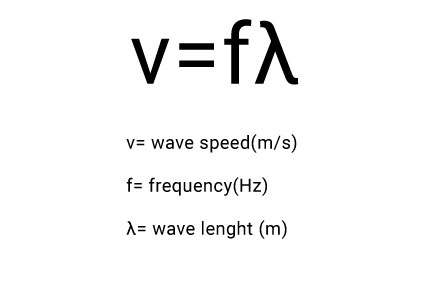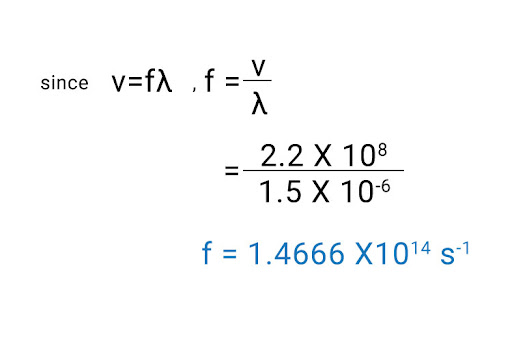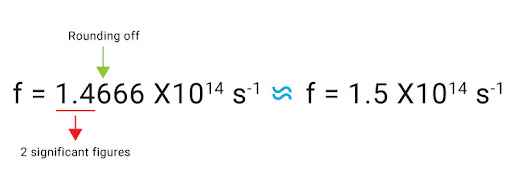# "An infared wave has a wavelength of 1.5 x10^–6 m. The speed of this wave is  2.2 × 10^8 m/s. Calculate the frequency of the wave. Give your answer in standard form and to 2 significant figures."

The infrared wave is the part of the electromagnetic spectrum that comes right before visible light (when ordered from low to high frequencies). It is a transverse progressive wave, and so to find the frequency of this infrared wave from its wavelength and speed, we have to use the equation for wave speed:From the question above, we have speed v = 2.2 x 108 m/s, and wavelength λ = 1.5 x 10-6 m. Therefore, the frequency f is:Upon using your scientific calculator, you’ll notice your answer contains a factor of 1014 - this is what we call the standard form, which is a neat way of writing very large numbers in a convenient form. All that’s left is to round this off to 2 significant figures: